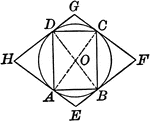### Circle With Inscribed and Circumscribed Quadrilaterals

Illustration showing a circle with an inscribed and circumscribed quadrilateral.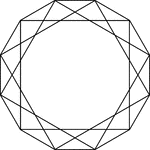### 3 Square Inscribed In A Dodecagon

Illustration of 3 squares inscribed in an regular dodecagon. Each vertex of the dodecagon is also a…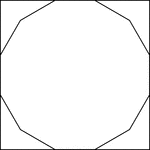### Square Circumscribed About A Dodecagon

Illustration of a square circumscribed about a regular dodecagon. This could also be described as a…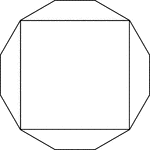### Square Inscribed In A Dodecagon

Illustration of a square inscribed in an regular dodecagon. This could also be described as a regular…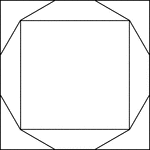### Squares Inscribed and Circumscribed About a Regular Dodecagon

Illustration of 2 squares; one inscribed in a regular dodecagon and the other circumscribed about the…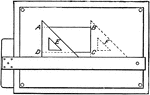### Drawing Rectangles

A triangle and a T-square can be used in conjunction to draw a rectangle.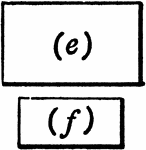### Similar Figures

2 rectangles that are similar figures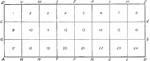### Fraction Boxes

Numbered boxes that are used for fractions. Fraction boxes within can be referred by the letter corners.…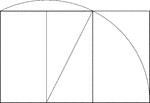### Construction Of A Golden Rectangle

Illustration showing the construction of a golden rectangle. Beginning with a unit square, a line is…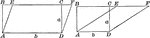### Area of a Parallelogram

Illustration of parallelogram AEFC drawn two different ways with base b and altitude/height a used to…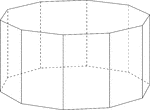### Decagonal Prism

Illustration of a decagonal prism with regular decagons for bases and rectangular faces. The hidden…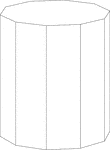### Decagonal Prism

Illustration of a right decagonal prism with regular decagons for bases and rectangular faces.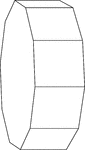### Decagonal Prism Resting On Its Side

Illustration of a right decagonal prism with regular decagons for bases and rectangular faces. The prism…### Swimming Pool Shaped Like A Decagonal Prism

Illustration of a swimming pool and water hose that is in the shape of a hollow regular decagonal prism…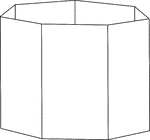### Heptagonal/Septagonal Prism

Illustration of a hollow right heptagonal/septagonal prism with regular heptagons/septagons for bases…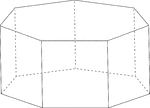### Heptagonal/Septagonal Prism

Illustration of a regular right heptagonal/septagonal prism with regular heptagons/septagons for bases…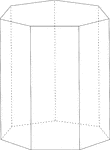### Heptagonal/Septagonal Prism

Illustration of a right heptagonal/septagonal prism with regular heptagons/septagons for bases and rectangular…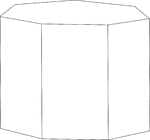### Heptagonal/Septagonal Prism

Illustration of a right heptagonal/septagonal prism with regular heptagons/septagons for bases and rectangular…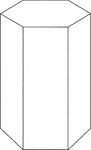### Hexagonal Prism

Illustration of a right hexagonal prism.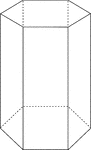### Hexagonal Prism

Illustration of a right hexagonal prism with hidden edges shown.### Hexagonal Prism

Illustration of a right hexagonal prism with a height less than the length of the edge of the hexagon.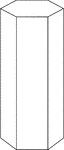### Hexagonal Prism

Illustration of a right hexagonal prism with a height greater than the length of the edge of the hexagon.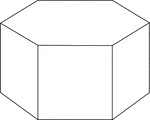### Hexagonal Prism

Illustration of a right hexagonal prism with hexagons for bases and square faces.### Hexagonal Prism

Illustration of a right hexagonal prism with hexagons for bases and square faces. The hidden edges are…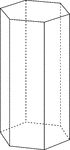### Hexagonal Prism

Illustration of a right hexagonal prism with hexagons for bases and rectangular faces. The height of…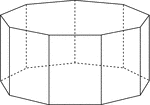### Nonagonal Prism

Illustration of a right nonagonal prism with regular nonagons for bases and rectangular faces. The hidden…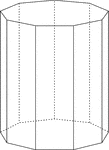### Nonagonal Prism

Illustration of a right nonagonal prism with regular nonagons for bases and rectangular faces. The height…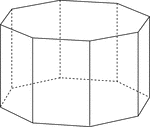### Octagonal Prism

Illustration of a right octagonal prism with regular octagons for bases and rectangular faces. The hidden…### Octagonal Prism

Illustration of a right octagonal prism with regular octagons for bases and rectangular faces. The height…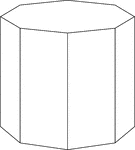### Octagonal Prism

Illustration of a right octagonal prism with octagons for bases and rectangular faces. The height of…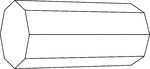### Octagonal Prism Resting On Side

Illustration of a right octagonal prism, with octagons for bases and rectangular faces, that is resting…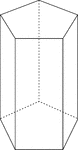### Pentagonal Prism

Illustration of a right pentagonal prism with regular pentagons for bases and rectangular faces. The…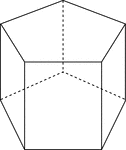### Pentagonal Prism

Illustration of a right pentagonal prism with regular pentagons for bases and rectangular faces. The…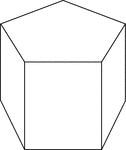### Pentagonal Prism

Illustration of a right pentagonal prism with regular pentagons for bases and rectangular faces. The…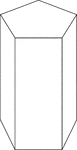### Pentagonal Prism

Illustration of a right pentagonal prism with regular pentagons for bases and rectangular faces. The…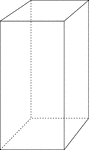### Right Rectangular Prism

Illustration of a right rectangular prism with the height greater than the length and width. The bases…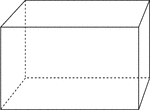### Right Rectangular Prism

Illustration of a right rectangular prism. The bases are congruent rectangles and the opposite faces…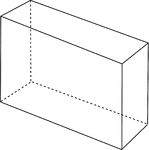### Right Rectangular Prism

Illustration of a right rectangular prism that is viewed at an angle. The bases are congruent rectangles…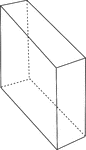### Right Rectangular Prism

Illustration of a right rectangular prism that is viewed at an angle. The bases are congruent rectangles…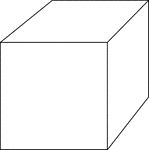### Right Rectangular Prism

Illustration of a right rectangular prism. The bases are congruent rectangles and the opposite faces…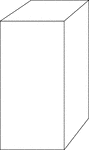### Right Rectangular Prism

Illustration of a right rectangular prism with the height greater than the length and width. The bases…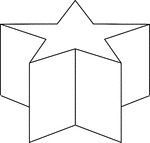### Star-Shaped Decagonal Prism

Illustration of a non-regular decagonal prism in the shape of a star. Then ends/bases are made of star-shaped…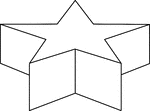### Star-Shaped Decagonal Prism

Illustration of a non-regular decagonal prism in the shape of a star. Then ends/bases are made of star-shaped…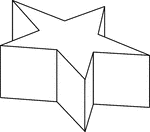### Star-Shaped Decagonal Prism

Side view of a non-regular decagonal prism in the shape of a star. Then ends/bases are made of star-shaped…### 2 Octagonal Prisms

Illustration of 2 right octagonal prisms with congruent bases, but different heights. The height of…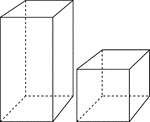### 2 Rectangular Prisms

Illustration of 2 right rectangular prisms. The bases are congruent, but the height of the smaller prism…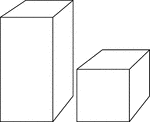### 2 Rectangular Prisms

Illustration of 2 right rectangular prisms. The bases are congruent, but the height of the smaller prism…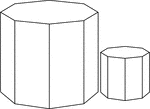### 2 Similar Octagonal Prisms

Illustration of 2 Similar right octagonal prisms. The height and length of the edges of the smaller…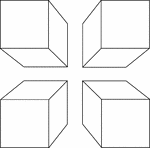### 4 Congruent Rectangular Prisms

Illustration of 4 congruent rectangular prisms placed in the shape of a square. They are arranged to…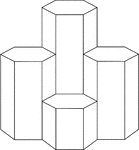### 4 Hexagonal Prisms

A cluster of 4 right hexagonal prisms with congruent bases, but varying heights.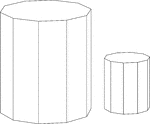### Similar Decagonal Prisms

Illustration of 2 similar right decagonal prisms. Both have regular decagons for bases and rectangular…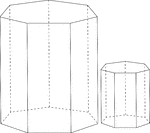### Similar Heptagonal/Septagonal Prisms

Illustration of 2 similar right heptagonal/septagonal prisms. Both have regular heptagons/septagons…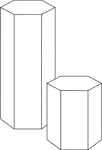### Similar Hexagonal Prisms

Illustration of 2 similar right hexagonal prisms. The height of the prism and length of the side of…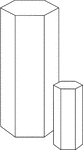### Similar Hexagonal Prisms

Illustration of 2 similar right hexagonal prisms. The height of the prism and length of the side of…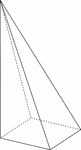### Skewed Rectangular Pyramid

Illustration of a non-right, or skewed, rectangular pyramid with hidden edges shown. The base is a rectangle…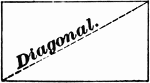### Rectangle

A rectangle is a parallelogram whose angles are all right angles.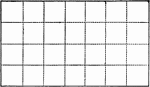### Area of a Rectangle

Rectangle divided into squares to demonstrate area.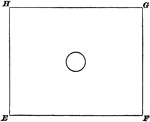### Circle Inside Rectangle

Illustration of a circle inside of a rectangle. The two figures have the same center.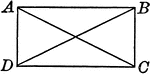### Rectangle With Diagonals

Illustration to show that if the diagonals of a parallelogram are equal, the figure is a rectangle.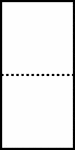### Divided Rectangle

A rectangle divided evenly by a dotted line.